# Kraken Daily Market Report for June 14 2020

## Overview

• Total trading volume at $71 million. Bitcoin prices were stable throughout the day, except for a quick price drop and return around 22:00 UTC. • Fiat trading dominated by USD with 66% of the fiat trading volume. • PAXG and a few Stablecoins had small positive returns, all other coins except one had losses against USD. The exception was Melon (MLN) +2.3%, which continues an impressive month. Melon also has the largest week-over-week increase in trading volume. June 14, 2020$71.0M traded across all markets today
Crypto, EUR, USD, JPY, CAD, GBP, CHF
XBT
$9332.3 ↓1.5%$44.4M
ETH
$231.74 ↓2.6%$13.8M
USDT
$0.9993 ↓0.05%$3.72M
BCH
$237.65 ↓1.3%$1.43M
XRP
$0.1900 ↓1.2%$1.3M
XTZ
$2.5867 ↓4.1%$1.16M
DAI
$1.0143 ↑1.4%$1.12M
$0.0763 ↓3.5%$899K
LTC
$44.034 ↓2.3%$713K
$3.9327 ↓4.4%$638K
REP
$15.480 ↓8.4%$626K
MLN
$6.5015 ↑2.3%$595K
ATOM
$2.7520 ↓4.0%$507K
XLM
$0.0706 ↓4.6%$490K
SC
$0.0030 ↓4.6%$364K
USDC
$0.9998 ↑0.01%$358K
NANO
$1.0462 ↓8.0%$323K
BAT
$0.2236 ↓3.8%$274K
XMR
$65.345 ↓1.7%$263K
EOS
$2.5655 ↓1.5%$240K
ALGO
$0.2333 ↓0.5%$229K
WAVES
$1.1775 ↓5.7%$205K
ETC
$6.3152 ↓2.8%$180K
DASH
$72.517 ↓2.3%$136K
PAXG
$1734.6 ↑0.29%$118K
ICX
$0.3220 ↓4.4%$108K
ZEC
$51.015 ↓3.4%$98.2K
OMG
$1.5626 ↓3.5%$97.1K
QTUM
$1.7196 ↓3.3%$80.2K
TRX
$0.0161 ↓3.2%$66.7K
OXT
$0.1805 ↓2.3%$64.8K
GNO
$28.909 ↓5.1%$48.5K
LSK
$1.2031 ↓2.5%$30.5K
XDG
$0.0024 ↓0.7%$8.79K

#####################. Trading Volume by Asset. ##########################################

The figures below break down the trading volume of the largest, mid-size, and smallest assets. Cryptos are in purple, fiats are in blue. For each asset, the chart contains the daily trading volume in USD, and the percentage of the total trading volume. The percentages for fiats and cryptos are treated separately, so that they both add up to 100%.

Figure 1: Largest trading assets: trading volume (measured in USD) and its percentage of the total trading volume (June 14 2020)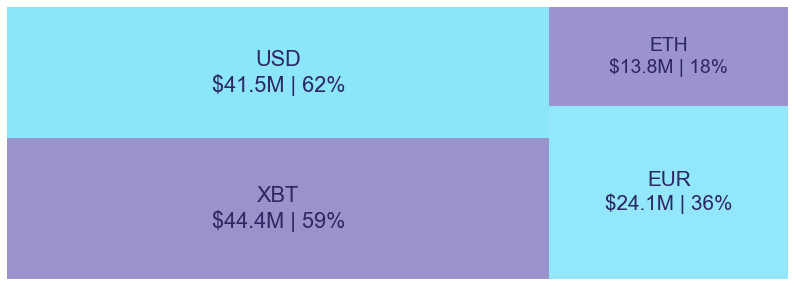Figure 2: Mid-size trading assets: (measured in USD) (June 14 2020)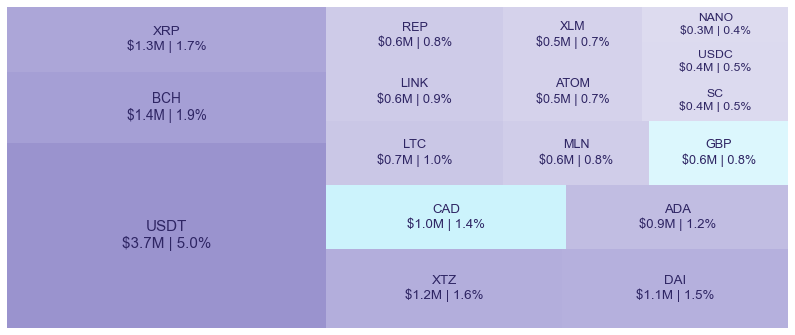Figure 3: Smallest trading assets: (measured in USD) (June 14 2020)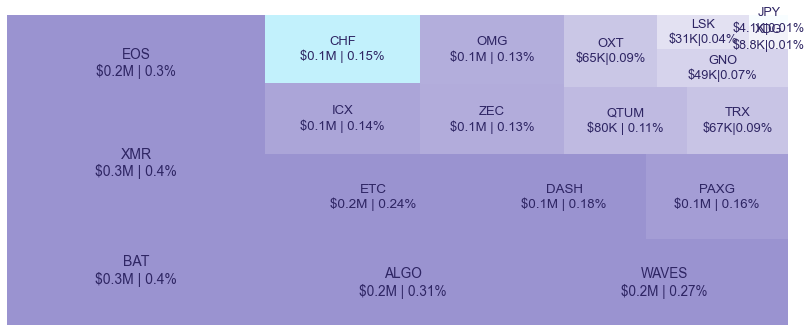Spread percentage is the width of the bid/ask spread divided by the bid/ask midpoint. The values are generated by taking the median spread percentage over each minute, then the average of the medians over the day.

Figure 4: Average spread % by pair (June 14 2020)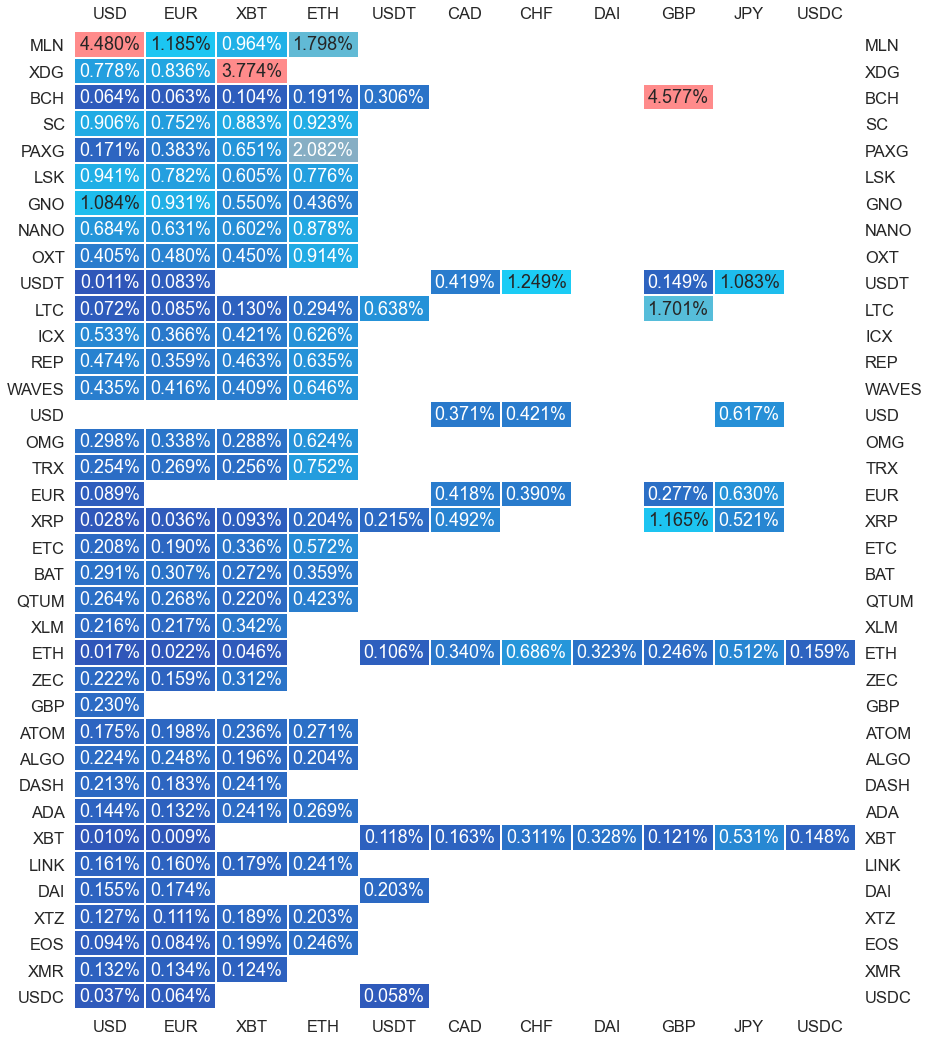.

#########. Returns and Volume ############################################

## Returns and Volume

Figure 5: Returns of the four highest volume pairs (June 14 2020)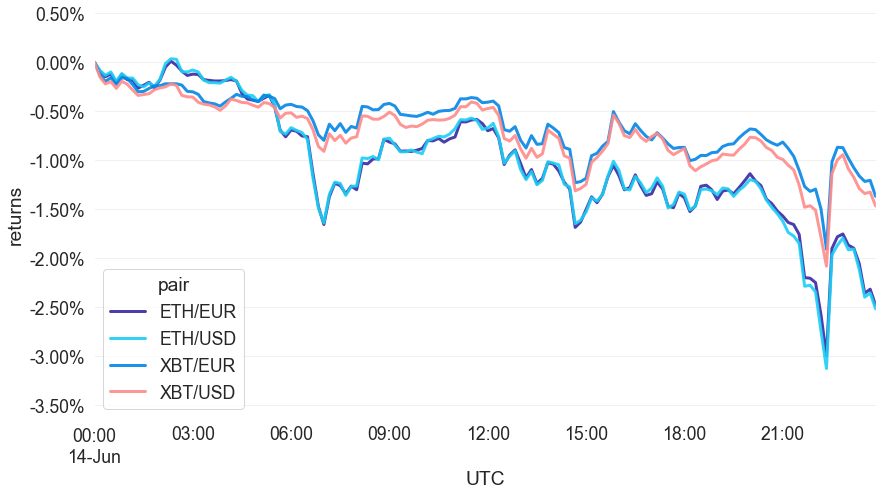Figure 6: Volume of the major currencies and an average line that fits the data to a sinusoidal curve to show the daily volume highs and lows (June 14 2020)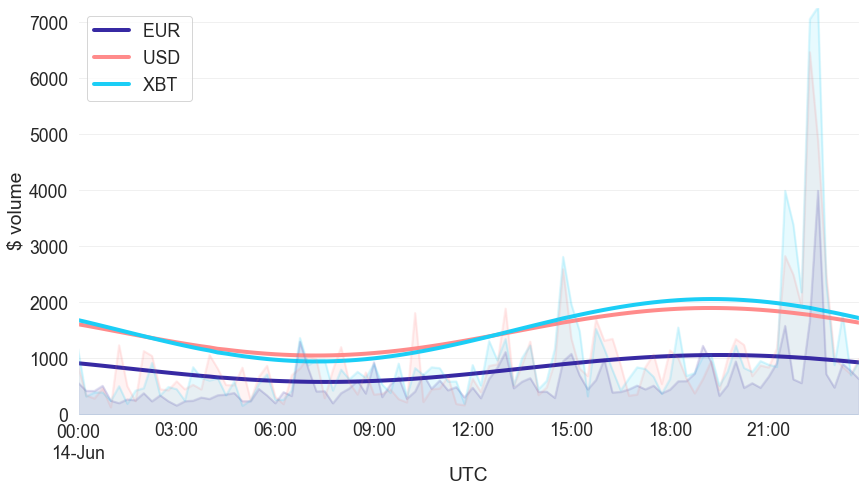###########. Daily Returns. #################################################

## Daily Returns %

Figure 7: Returns over USD and XBT. Relative volume is indicated by the size of the font. (June 14 2020)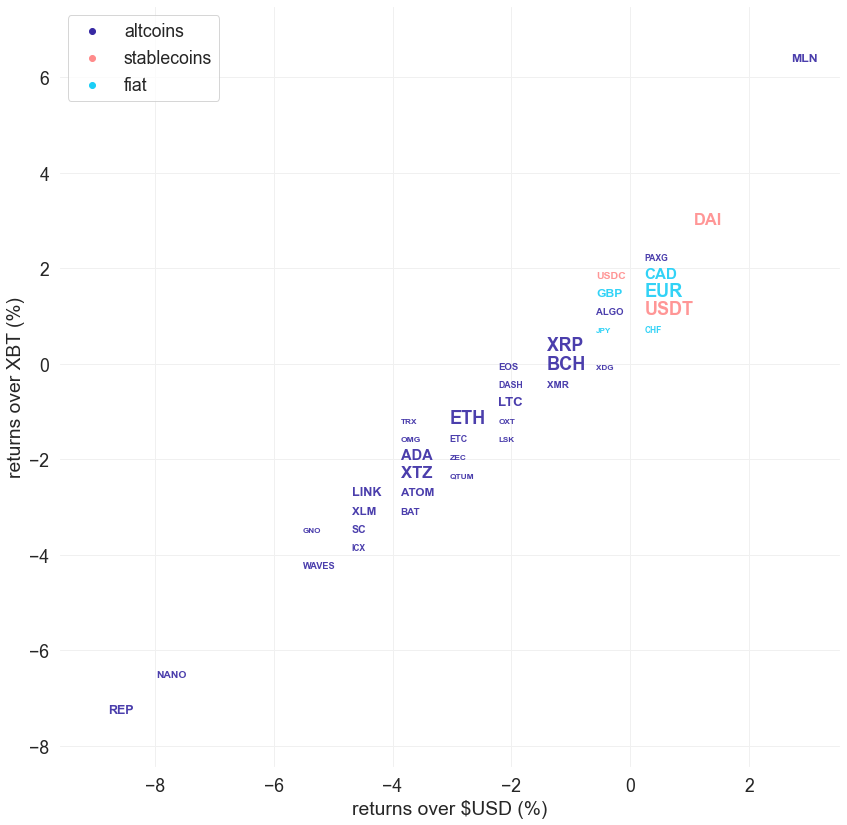###########. Disclaimer #################################################

The values generated in this report are from public market data distributed from Kraken WebSockets api. The total volumes and returns are calculated over the reporting day using UTC time.